﻿ Identification of Spatial Distribution of Geochemical Anomalies Based on GIS and C-A Fractal Model – A Case Study of Jiurui Copper Mining Area

### Identification of Spatial Distribution of Geochemical Anomalies Based on GIS and C-A Fractal Model –...

Anh Huy Hoang, Tien Thanh Nguyen

Journal of Geosciences and Geomatics

## Identification of Spatial Distribution of Geochemical Anomalies Based on GIS and C-A Fractal Model – A Case Study of Jiurui Copper Mining Area

Anh Huy Hoang1, Tien Thanh Nguyen1,1Hanoi University of Natural Resources and Environment, Hanoi, Vietnam

### Abstract

Identification of spatial distribution of geochemical anomalies for mineral exploration is a fundamantal issue in the field of exploration geochemistry. Conventional methods are hard to identify geochemical anomalies due to extreme values. In this study, C-A fractal model is first applied to identify anomalies, GIS is then used to visualized the results. 1482 geochemical analytical data of Cu ore-forming element from Jiurui copper mining area are used as as one experiment. The results show that this method can effectively identify spatial distribution of geochemical anomalies. These anomalous areas can be used to interpret possible origins of mineralization, which is agreement with petrological analysis and field survey results.

• Anh Huy Hoang, Tien Thanh Nguyen. Identification of Spatial Distribution of Geochemical Anomalies Based on GIS and C-A Fractal Model – A Case Study of Jiurui Copper Mining Area. Journal of Geosciences and Geomatics. Vol. 4, No. 2, 2016, pp 36-41. http://pubs.sciepub.com/jgg/4/2/3
• Hoang, Anh Huy, and Tien Thanh Nguyen. "Identification of Spatial Distribution of Geochemical Anomalies Based on GIS and C-A Fractal Model – A Case Study of Jiurui Copper Mining Area." Journal of Geosciences and Geomatics 4.2 (2016): 36-41.
• Hoang, A. H. , & Nguyen, T. T. (2016). Identification of Spatial Distribution of Geochemical Anomalies Based on GIS and C-A Fractal Model – A Case Study of Jiurui Copper Mining Area. Journal of Geosciences and Geomatics, 4(2), 36-41.
• Hoang, Anh Huy, and Tien Thanh Nguyen. "Identification of Spatial Distribution of Geochemical Anomalies Based on GIS and C-A Fractal Model – A Case Study of Jiurui Copper Mining Area." Journal of Geosciences and Geomatics 4, no. 2 (2016): 36-41.

 Import into BibTeX Import into EndNote Import into RefMan Import into RefWorks

12
Prev Next

### 1. Introduction

Spatial distribution identification of geochemical anomalies is a matter of great interest for geochemical exploration. In the 1960s, several procedures had been recommended for selecting threshold against which anomalies can be judged : (1) carry out an orientation survey to define a local threshold against which anomalies can be judged; (2) order the data and select the top 2½% of the data for further inspection if no orientation survey results are available; and (3) for large data sets, which cannot easily be ordered, use [MEAN±2SDEV] (SDEV: standard deviation) to identify about 2½% of upper (or lower) extreme values for further inspection and an underlying assumption that the data are drawn from a normal distribution . The data distribution is important and that must be known before doing anything else. But this basic requirement is still widely neglected although a number of papers and books address the problem [20, 23]. To avoid this problem extreme, i.e. ‘obvious’ outliers, are often removed from the data prior to the calculation . Another method is to first log-transform (log10 or ln) the data to minimize the influence of the outliers and then do the calculation . It is usually found that the transformed data do not follow a normal distribution [1, 2, 3, 4, 17, 19]. The widely-used classical statistical methods are likely to fail for strongly skewed data .  used robust statistics  and exploratory data analysis : the boxplot and the [median ± 2*mad] (mad: median absolute deviation) rule and empirical cumulative distribution functions for assisting in the estimation of threshold values and the range of background data.

Frequency analysis, such as histogram construction, Q-Q plots, probability plots, and box-plot have been commonly used for anomaly separation [14, 25, 26, 27, 28]. These non-spatial statistical methods ignore the spatial information or autocorrelation structure of the geochemical data. It is shown that the consideration of geometry and scale-independent properties of geochemical landscapes aims to accurately separate background and anomalies [7, 8, 9].  proposed the C-A fractal model. This model has been widely used to separate geochemical anomalies from background by considering both frequency distributions and spatial self-similar properties of geochemical variables [10, 11, 12, 14, 18, 29, 30].

In this study, spatial distribution of geochemical anomalies of Cu element will be identified by means of GIS and C-A fractal model with a case study of 1341 stream sediment samples with area of 8130 km2 and scale of 1:200.000 in Jiurui copper mining area in China.

### 2. Materials and Methods

2.1. Materials

According to the requirements of 1:200.000 regional stream sediment survey, a multi-element sediment geochemical survey of streams was carried out in the study area. A total of 1482 composite samples representing about 5364 km2 were collected. The sampling density was 1 composite sample per 4 km2. No samples were taken at grids where access was hard to obtain. There are more than 20 indexes in a composite sample, including Ag, As, Au, Be, Cd, Cu, Hg, Li, Mn, Mo, Nb, Pb, Sb, Sn, Th, V, W, Y, Zn, Al2O3, CaO, K2O, Na2O etc. There are two metallogenelic series in the study area. A total of 13 ore deposits were found marked by numeric characters from 1 to 13 (see Figure 1). Au, Ag and Cu are three ore-forming elements. Cu was chosen to identify geochemical anomalies in this study.Download asVeiw figureFigures index
Figure 1. Location map of 1482 stream sediment samples and 13 ore deposits
2.2. The Concentration-area Fractal Model

 proposed the concentration-area method by considering the spatial scale of the distribution of the data to separate geochemical anomalies from background. Contour maps can be used to obtain approximate relations between areas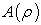and concentration values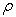, withdecreasing for increasing. Conversely, the area with concentration values less thanis an increasing function of. If the element concentration per unit area satisfies a fractal or multi-fractal model, then the areahas indeed a power-law type relation with. When the concentration per unit area follows a fractal model, this power-law relation has only one exponent. On the other hand, when the concentration per unit satisfies a multi-fractal model with a spectrum of fractal dimensions, then several separate power-law relations between areaandcan be established. This empirical model states that the area, enclosing concentration valueslesser or equal than a pre-defined threshold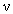, follow a power-law relation like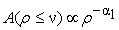(1)

Conversely, for areas with concentration valuesgreater than a pre-defined threshold, the relation becomes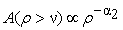(2)

In equations (1) and (2)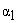and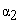represent characteristic exponents. Using multifractal theory  derived similar power-law equations. Therefore, for a range ofvalues close to its minimum, the predicted multi-fractal power-laws take the form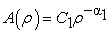(3)

and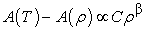(4)

where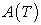is the total sampled area,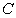and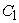are constants, andand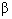are exponents associated with the maximum singularity exponent. For the range ofvalues close to its maximum, the equation obtained is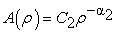(5)

where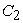is a constant, andis the exponent associated with the minimum singularity exponent. Therefore, assuming a multi-fractal model, equations (3), (4), (5) are equivalent to equation (1) and (2), and whenever a plot of logvs. logis obtained, values of the constants and exponents can be extracted. It is called concentration-area plot (C-A plot). The break in linearity of the experimental data points occurs for the value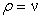corresponding to the threshold value for the anomalous area.

In the study, the data were first spatially interpolated to a fine (100×100) regular grid, increasing the number of data points so they can be used as a surrogate for measuring areas. The interpolated grid size was computed as [xmax - xmin]/ngrid, with a default value of 100 for ngrid. Akima's interpolation function was used to obtain a linear interpolation between the spatial data values [5, 6]. Then the interpolated values outside the survey area boundary were trimmed away, so there was no interpolation to points outside the survey area. The original and interpolated data together define the x-axis of the CP-plots. In the C-A plot, the y-axis shows the percentage of the interpolated values, plotted on a logarithmic scale, that are larger (or smaller) than each value plotted on a logarithmic scale on the x-axis. As the interpolated points are on a fine regular grid the number of points is a surrogate for the area of the interpolated map that is larger (or smaller) than the corresponding value on the x-axis. Thus the C-A plot displays the relationship between the percentage of the survey area that has a particular values and the actual value. Background levels will occur frequently and will represent the majority of the survey area, and low or high extreme values-containing areas will represent small percentages of the survey area. A single straight line indicates a single fractal relationship controlling the data distribution; multiple straight lines indicate multiple fractal processes controlling the data distribution. In order to reduce the influences of interpolation algorithm and data transformation on computing the C-A plot, the data were first Box-Cox transfromed so that extreme values and outliers do not over-influence the resulting interpolation, then simple triangulation was employed for the interpolation to avoid the data from being excessively smoothed as that will “smeer out” the very features being sought.

2.3. Data Treatment with Computer Softwares

The descriptive statistical parameters and exploratory data analysis plots were performed and conducted using the StatDA and MASS packages of Statistical Modeling & Computing - R Language (version i386 2.15.0) with the StatDA and MASS packages. Maps of spatial distribution of geochemical anomalies were produced with ArcGIS 9.3.

### 3. Results and Discussions

3.1. Cu Concentration in the Study Area

Table 1 provides information of Cu concentration. The mean value of Cu concentration in the study area was 37.33 μg/g, higher than the median value of 27.1 μg/g. The skewness of 15.8 indicates that Cu has an asymmetrical distribution with a long tail to the right and a positive skew. The kurtosis of 288.5 quantifies the shape of the data distribution does not match the normal distribution, a distribution is much more peaked than a normal distribution. Care must be taken in interpret ing the high values. It is likely the high values (or maximum values) of Cu concentration was caused by mineralization.

#### Table 1. Summary table of Cu concentration for original data and Box-Cox transformed dataDownload asPowerPoint Slide

Veiw figure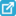View current table in a new window

The spatial distribution of Cu in the study area shows the influence of mineral deposits. There is a high value pattern of Cu in the north and the east. There are also some relatively high values scattered in the north-west where no deposits has so far detected showing the complexity or spatial heterogeneity of Cu concentration.

The distribution of the Cu is far from symmetrical. For Box-Cox transformed data, the mean value is equivalent to the median value, which shows that transformed Cu concentration is evenly divided around the mean. The kurtosis of -0.156 is close to zero (greater than -1.0 and less than 1.0), indicating that the distribution of the transformed data is close to symmetrical.

Figure 2 shows the distribution of the variable Cu. The histograms show that the distribution of the “raw” data are strongly right-skewed, many very high values dominate the plot (Figure 2a). The distances of these high values are far from the main body of the data. The distribution of original data is not normal but extremely right skewed, as a result typical asymmetrical, sigmoidal S-shapes are not present in the ECDF-plot (Figure 2b). To reduce the influence of the outliers and to obtain much information of value about the shape of the distribution, the data were scaled by using Box-Cox transformation. The Box-Cox transformation results in an almost symmetrical distribution of the strongly right skewed original data. The histogram and density trace still show a slight right skew (Figure 2d). The ECDF-plots begin to display an S-shape (Figure 2a), the right skew is however still clearly reflected.Download asVeiw figureFigures index
Figure 2. Histogram, density trace, 1-D scatter plot, boxplot and ECDF-plot of Cu concentration for raw data (a, b) and Box-Cox transformed data (c, d)
3.2. Spatial Distribution of Anomalies

A display of four panels was shown in Figure 3. The percentage cumulative probability (CPP) plot of the data in the upper left and the CP plot of the interpolated data to be used in the C-A plot in the upper right. The lower left panel contains an image of the interpolated data, and the lower right the C-A plot. The distribution of Cu concentration is positively skewed, therefore, the data were first log-transformed so that extreme values do not over-influence the resulting interpolation. 1341 sampling points were used to generate 8996 grids after outside the survey area boundary were trimmed away. The image of the interpolated values were displayed with low values being plotted in blue, and as the interpolated values increase they take on green, yellow and orange colors.

The C-A plots in Figure 3d and Figure 4b were prepared by ordering the interpolated values from the maximum values downwards. It can be seen that the data are spatially multi-fractal, different multiple populations that are spatially dependent are present in the data set. A break at 48 μg/g of the straight line represented a threshold that was used to classify the Cu data set into background and anomalous populations. Other breaks indicating the presence of at least five populations in the Cu data: low background (≤ 18 μg/g), high background (18 - 48 μg/g), low anomaly (48 - 200 μg/g), high anomaly (200 - 500 μg/g) and very high anomaly (≥ 500 μg/g). It is apparent that the data do not follow a single fractal relationship, with another relationship present at low levels and a more complex relationship above 200 about μg/g Cu near the smelters. To focus on the lower end of the fractal distribution, the interpolated values from lowest to highest were ordered to achieve C-A plot see Figure 4a. It is immediately apparent that there are three major fractal processes present: one is very low background below about 10 μg/g; one between 10 μg/g and 48 μg/g; one above 48 μg/g. Figure 4 shows spatial distributions of different background levels and anomaly levels of the Cu data based on thresholds defined from concentration-area fractal method. Very low to low background Cu values are distributed in the southern (lower half-part) part of the study area (Figure 5). High background Cu values are mostly distributed in the south, west and south-western parts. Low anomaly Cu values are mostly distributed in the north, east, north-west and some in the south-western parts. High anomaly and very high anomaly Cu values are distributed in the northern part and north-eastern part where known ore deposits were located.Download asVeiw figureFigures index
Figure 3. The CP-plots of the original data (a) and of the interpolated data (b), the grey-scale map of the interpolated data (c) and the C-A plotfor log-transformed Cu (d)Download asVeiw figureFigures index
Figure 4. The C-A plots for Cu: cumulated upwards (a) and downwards (b)Download asVeiw figureFigures index
Figure 5. Spatial distribution map of background and anomalous samples for Cu

### 4. Conclusions

Geochemical anomalies provide significant ore-finding information. The identification of anomalous areas is thus an important part of data processing. In this study, geochemical anomalies were identified by means of GIS and C-A multifractal model. This method overcomes the distortion effects of outliers on the conventional methods. The results show that outlier areas accord the objective reality and have a good conformity with known deposits in Jiurui copper mining area. Two important issues can be concluded: (1) Geochemical anomalies might distort the analysis to a great degree, it is therefore suggested to do the data transformation before the analysis. (2) Geochemical data are spatially multi-fractal, C-A model can effectively be used to separate and identify different multiple populations especially extreme values or anomalies.

### Acknowledgments

The authors thank reviewers for assistance with suggested improvements to this manuscript and Dr. Peng Gong (formerly of China University of Geosciences) for the data collection.

### References

  Ahrens, L., 1953. A fundamental law of geochemistry. Nature, 172, 1148.In article View Article  Ahrens, L., 1954a. The lognormal distribution of the elements (a fundamental law of geochemistry and its subsidiary). Geochim Cosmochi Acta, 5, 49-74.In article View Article  Ahrens, L., 1954b. The lognormal distribution of the elements II. Geochim Cosmochi Acta, 6, 121-132.In article View Article  Ahrens, L., 1957. Lognormal-type distribution III. Geochim Cosmochi Acta, 11, 205-213.In article View Article  Akima, H., 1978. A Method of Bivariate Interpolation and Smooth Surface Fitting for Irregularly Distributed Data Points. ACM Transactions on Mathematical Software, 4(2), 148-164.In article View Article  Akima, H., 1996. Algorithm 761: scattered-data surface fitting that has the accuracy of a cubic polynomial. ACM Transactions on Mathematical Software, 22(3), 362-371.In article View Article  Carranza, E., 2009. Geochemical anomaly and mineral prospectivity mapping in GIS in Handbook of Exploration and Environmental Geochemistry. Amsterdam: Elsevier.In article PubMed  Cheng, Q.M., Agterberg F., Ballantyne, S., 1994. The separation of geochemical anomalies from background by fractal methods. Journal of Geochemical Exploration, 51(2), 109-130.In article View Article  Cheng, Q.M., 1999. Spatial and scaling modelling for geochemical anomaly separation. Journal of Geochemical Exploration, 65(3), 175-194.In article View Article  Cheng, Q.M, Li Q., Xu Y., 2000. Self-similarity recognition and geochemical anomaly separation. In Proceedings of Geological Association of Canada Meeting (GeoCanada 2000). Calgary: University of Calgary.In article  Cheng, Q.M., 2004. A new model for quantifying anisotropic scale variance and for decomposition of mixing patterns. Mathematical Geology, 36(3), 345-360.In article View Article  Cheng, Q.M., 2007. Mapping singularities with stream sediment geochemical data for prediction of undiscovered mineral deposits in Gejiu, Yunnan Province, China. Ore Geology Reviews, 32(1), 314-324.In article View Article  Cheng, Q.M., Agterberg, F., 2009. Singularity analysis of ore-mineral and toxic trace elements in stream sediments. Computers & Geosciences, 35(2), 234-244.In article View Article  Garrett, R., 1989. A cry from the heart. Explore, 66:18-20In article  Hawkes, H., and Webb, J., 1962. Geochemistry in mineral exploration. New York: Harper.In article PubMed  Huber, P., 1981. Robust statistics. New York: Wiley & Sons.In article View Article  McGrath, S. and Loveland, P., 1992. The Soil Geochemical Atlas of England and Wales. London: Blackie.In article  Li, Q.M. and Cheng, Q.M., 2004. Fractal singular-value (eigen-value) decomposition method for geophysical and geochemical anomaly reconstruction. Earth Science - Journal of China University of Geosciences, 29(1),109-118.In article  Nguyen, T.T., et al., 2013. Robust statistics and EDA techniques for identification of the geochemical anomalies. Computing Techniques for Geophysical and Geochemical Exploration, 35(3), 307-113.In article  Philip, G.M. and Watson, D.F., 1987. Probabilism in geological data analysis. Geological Magazine, 1987, 124(6), 577-583.In article View Article  Reimann, C. and Filzmoser, P., 2000. Normal and lognormal data distribution in geochemistry: death of a myth. Consequences for the statistical treatment of geochemical and environmental data. Environmental geology, 39(9), 1001-1014.In article View Article  Reimann, C., Filzmoser P. and Garrett, R.G., 2005. Background and threshold: critical comparison of methods of determination. Science of the Total Environment, 346(1-3), 1-16.In article View Article  PubMed  Rock, N., 1988. Numerical geology. New York Berlin Heidelberg: Springger Verlag.In article  Tukey, J., 1977. Exploratory Data Analysis. Reading: Addison-Wesley.In article  Sinclair, A.J., 1974. Selection of threshold in geochemical data using probability graphs. J. Geochem. Explor. 3,129-149.In article View Article  Sinclair, A.J., 1976. Application of probability graphs in mineral exploration. Assoc. Explor. Geochem. 4, 95.In article  Sinclair, A.J., 1991. A fundamental approach to threshold estimation in exploration geochemistry. Probability plots revisited. J. Geochem. Explor. 41,1-22.In article View Article  Stanley, C.R. and Sinclair, A.J., 1989. Comparison of probability plots and gap statistics in the selection of threshold for exploration geochemistry data. J. Geochem. Explor., 32, 355-357.In article View Article  Xiao, F. and Chen, J.G., 2012. Fractal projection pursuit classification model applied to geochemical survey data. Computers & Geosciences, 45(10-11), 75-81.In article View Article  Xu, Y.G., Cheng, Q.M., 2001. A fractalfiltering technique for processing regional geochemical maps for mineral exploration. Journal of Geochemistry: Exploration, Environment, Analysis, 1(2): 147-156.In article